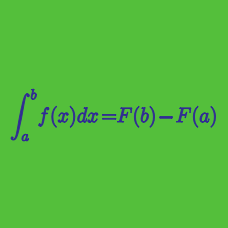Calculus

# Basic Properties of Integrals

If $\int_0^1 f(x) \, dx = 4$, what is the value of

$\int_0^1 2 f(x) \, dx ?$

If $\int_0^1 f(x) \, dx = 2$ and $\int_0^1 g(x) \, dx = 6$, what is the value of

$\int_0^1 \left( 6 f(x) + 2 g(x) \right) \, dx ?$

If $\int_0 ^ {4} f(x) \, dx = 3$, what is the value of

$\int_0^{4} \left( 3 - f(x) \right) \, dx ?$

If $\int_0^{10} f(x) \, dx = 27$ and $\int_0^5 f(x) \, dx = 7$, then what is the value of

$\int_5^{10} f(x) \, dx ?$

Suppose $f(x)$ is an odd function and $g(x)$ is an even function such that

$\int_0 ^ {5} f(x) \, dx = 7 \hspace{.6cm} \int_{5}^{15} f(x) \, dx = 8 \\ \int_{-5}^{0} g(x) \, dx = 1 \hspace{.6cm} \int_{5}^{15} g(x) \, dx = 2$

What is the value of $\int_{-5}^{15} \left( f(x) + g(x) \right) \, dx ?$

×